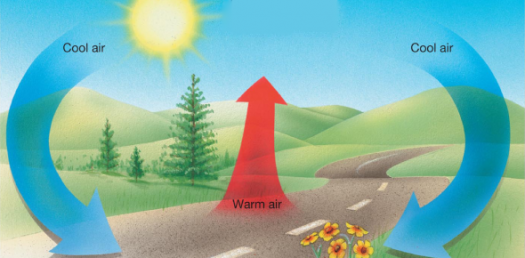# Heat Transfer Quiz! Trivia Questions

10 Questions | Total Attempts: 3687SettingsHeat Transfer is the transmission of thermal energy due to a gradient in temperature. Do you know which materials are a great conductor of heat and which ones are not? The biggest example of heat energy in our solar system is the sun itself as it radiates heat to warm the planet. This type of energy can be converted from other types of energy. Try answering the trivia questions in this heat transfer quiz.

• 1.
Temperature is a measure of ...
• A.

Total kinetic energy

• B.

Average potential energy

• C.

Average kinetic energy

• D.

Kinetic energy plus potential energy

• 2.
The lowest possible temperature is ...
• A.

0 degrees Kelvin

• B.

-273 degrees Fahrenheit

• C.

0 degrees Fahrenheit

• D.

-273 degrees Kelvin

• 3.
A thermometer works because the liquid in it ___ when heated.
• A.

Contracts

• B.

Becomes solid

• C.

Becomes elastic

• D.

Expands

• 4.
Heat is thermal energy that is transferred from one object to another because of a difference in what?
• A.

Mass

• B.

Temperature

• C.

Volume

• D.

Potential energy

• 5.
Fast food restaurants keep food warm by placing them under heat lamps. These lamps transfer heat by what means?
• A.

Convection

• B.

Conduction

• C.

• D.

Condensation

• 6.
When you burn your finger by touching a hot skillet, the heat is transferred to your finger by ...
• A.

Convection

• B.

Conduction

• C.

• D.

Condensation

• 7.
The purpose of putting a cold beverage into an insulated container is to ...
• A.

Cause the transfer of heat

• B.

Prevent the transfer of heat

• C.

Increase the liquid's thermal energy

• D.

Decrease the liquid's thermal energy

• 8.
Heat is thermal energy that is ...
• A.

Getting warmer

• B.

Getting cooler

• C.

Stationary

• D.

Flowing from one object to another

• 9.
Which one of the following is a good insulator?
• A.

Gold

• B.

Iron

• C.

Cotton

• D.

Copper

• 10.
Which one of the following is the best conductor?
• A.

Aluminum

• B.

Cotton

• C.

Wood

• D.

Plastic

Related TopicsBack to top